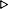ToC DocOverview CGDoc RelNotes Index PermutedIndex
Allegro CLANSI Common Lisp3 Evaluation and Compilation3.8 Dictionary Evaluation and Compilation

 3.8.20 dynamic-extent Declaration

Syntax:
(dynamic-extent [[{var}* | (function fn)*]])

Arguments:
var - a variable name.

fn - a function name.

Valid Context:
declaration

Binding Types Affected:
variable, function

Description:

In some containing form, F, this declaration asserts for each vari (which need not be bound by F), and for each value vij that vari takes on, and for each object xijk that is an otherwise inaccessible part of vij at any time when vij becomes the value of vari, that just after the execution of F terminates, xijk is either inaccessible (if F established a binding for vari) or still an otherwise inaccessible part of the current value of vari (if F did not establish a binding for vari). The same relation holds for each fni, except that the bindings are in the function namespace.

The compiler is permitted to use this information in any way that is appropriate to the implementation and that does not conflict with the semantics of Common Lisp.

dynamic-extent declarations can be free declarations or bound declarations.

The vars and fns named in a dynamic-extent declaration must not refer to symbol macro or macro bindings.

Examples:
Since stack allocation of the initial value entails knowing at the object's creation time that the object can be stack-allocated, it is not generally useful to make a dynamic-extent declaration for variables which have no lexically apparent initial value. For example, it is probably useful to write:

``` (defun f ()
(let ((x (list 1 2 3)))
(declare (dynamic-extent x))
...))
```

This would permit those compilers that wish to do so to stack allocate the list held by the local variable x. It is permissible, but in practice probably not as useful, to write:

``` (defun g (x) (declare (dynamic-extent x)) ...)
(defun f () (g (list 1 2 3)))
```

Most compilers would probably not stack allocate the argument to g in f because it would be a modularity violation for the compiler to assume facts about g from within f. Only an implementation that was willing to be responsible for recompiling f if the definition of g changed incompatibly could legitimately stack allocate the list argument to g in f.

Here is another example:

``` (declaim (inline g))
(defun g (x) (declare (dynamic-extent x)) ...)
(defun f () (g (list 1 2 3)))

(defun f ()
(flet ((g (x) (declare (dynamic-extent x)) ...))
(g (list 1 2 3))))

```
In the previous example, some compilers might determine that optimization was possible and others might not.

A variant of this is the so-called "stack allocated rest list" that can be achieved (in implementations supporting the optimization) by:

``` (defun f (&rest x)
(declare (dynamic-extent x))
...)
```

Note that although the initial value of x is not explicit, the f function is responsible for assembling the list x from the passed arguments, so the f function can be optimized by the compiler to construct a stack-allocated list instead of a heap-allocated list in implementations that support such.

In the following example,

``` (let ((x (list 'a1 'b1 'c1))
(y (cons 'a2 (cons 'b2 (cons 'c2 nil)))))
(declare (dynamic-extent x y))
...)
```
The otherwise inaccessible parts of x are three conses, and the otherwise inaccessible parts of y are three other conses. None of the symbols a1, b1, c1, a2, b2, c2, or nil is an otherwise inaccessible part of x or y because each is interned and hence accessible by the package (or packages) in which it is interned. However, if a freshly allocated uninterned symbol had been used, it would have been an otherwise inaccessible part of the list which contained it.

```;; In this example, the implementation is permitted to stack allocate
;; the list that is bound to X.
(let ((x (list 1 2 3)))
(declare (dynamic-extent x))
(print x)
:done)(1 2 3):DONE

;; In this example, the list to be bound to L can be stack-allocated.
(defun zap (x y z)
(do ((l (list x y z) (cdr l)))
((null l))
(declare (dynamic-extent l))
(prin1 (car l))))ZAP
(zap 1 2 3)123NIL

;; Some implementations might open-code LIST-ALL-PACKAGES in a way
;; that permits using stack allocation of the list to be bound to L.
(do ((l (list-all-packages) (cdr l)))
((null l))
(declare (dynamic-extent l))
(let ((name (package-name (car l))))
(when (string-search "COMMON-LISP" name) (print name))))"COMMON-LISP""COMMON-LISP-USER"NIL

;; Some implementations might have the ability to stack allocate
;; rest lists.  A declaration such as the following should be a cue
;; to such implementations that stack-allocation of the rest list
;; would be desirable.
(declare (dynamic-extent x))
(apply #'+ x))ADD
(add 1 2 3)6

(defun zap (n m)
;; Computes (RANDOM (+ M 1)) at relative speed of roughly O(N).
;; It may be slow, but with a good compiler at least it
;; doesn't waste much heap storage.  :-}
(let ((a (make-array n)))
(declare (dynamic-extent a))
(dotimes (i n)
(declare (dynamic-extent i))
(setf (aref a i) (random (+ i 1))))
(aref a m)))ZAP
(< (zap 5 3) 3)true
```

The following are in error, since the value of x is used outside of its extent:

``` (length (list (let ((x (list 1 2 3)))  ; Invalid
(declare (dynamic-extent x))
x)))

(progn (let ((x (list 1 2 3)))  ; Invalid
(declare (dynamic-extent x))
x)
nil)
```